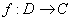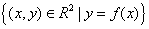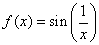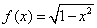## Preliminary test on functions and limits

### Gap-fill exercise

Write the correct answers. Every gap must be filled by one only word. When finished press Check. Pressing the Hint button adds the next correct letter in the answer.

1 - Given a function, D is called the of the function and C is the .
2 - The subset f(D) of C is called the of the function.
3 - If f(D)=C the function is called .
4 - If f(x1) = f(x2) only when x1 = x2 the function is called .
5 - A function is called bijective if it is both and injective.
6 - A function like f(x)=sign(x) is a function defined in .
7 - A function like f(x)=sign(x) is a non- function.
8 - The setis called the of the function.
9 - An interval like [a,b] is a interval.
10 - An interval like ]a,b[ is an interval.
11 - The real line with the two new elements +∞ and -∞ is called the real line.
12 - The limit is the of a function as x gets to a given point c of the domain.
13 - A point in whose neighbourhoods we can always find other of a set A is called an point for A.
14 - As x tends to the functionhas no limit.
15 - It makes no sense to calculate the limit ofas x tends to 2 because 2 is far from the , or, better, 2 is not an point for the .